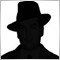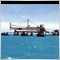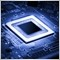• Overview
• Reviews (6)

# ImpAlligator

The standard indicator Alligator is calculated on the basis of smoothed moving average and median price.

This modification allows calculating the indicator on the basis of any combination of 4 basic prices: Close, Open, High, and Low, and choosing one of 6 methods of averaging: simple (SMA), exponential (EMA), smoothed (SMMA), linear weighted (LWMA), double exponential (DEMA), triple exponential (TEMA).

Input parameters:

• Jaws period - period of Alligator's jaws;
• Jaws shift - shift of Alligator's jaws;
• Teeth period - period of Alligator's teeth;
• Teeth shift - shift of Alligator's teeth;
• Lips period - period of Alligator's lips;
• Lips shift - shift of Alligator's lips;
• Moving average mode - averaging method: Simple (SMA), Exponential (EMA), Smoothed (SMMA), Linear Weighted (LWMA), Double Exponential (DEMA), Triple Exponential (TEMA);
• Close - weight coefficient of the CLOSE price for calculation of the individual price;
• Open - weight coefficient of the OPEN price for calculation of the individual price;
• High - weight coefficient of the HIGH price for calculation of the individual price;
• Low - weight coefficient of the LOW price for calculation of the individual price.

Example 1. Calculate indicator by OPEN price: Close=0, Open=1, High=0, Low=0.
Example 2. Calculate indicator by WEIGHTED price: Close=2, Open=0, High=1, Low=1.
Example 3. Calculate indicator by individual price constant: Close=6, Open=7, High=1, Low=3. Price=(6*Close + 7*Open + 1*High + 3*Low)/17.16477
Nikolay Gaylis 2019.01.14 08:04

User didn't leave any comment to the rating12010
2018.02.01 11:09

User didn't leave any comment to the rating5611
2017.11.09 14:53

User didn't leave any comment to the rating6158
2017.07.21 17:30

User didn't leave any comment to the rating7173
2016.03.14 04:26

User didn't leave any comment to the rating7826
2014.04.08 12:32

User didn't leave any comment to the rating